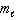# Problem: The quantum number ______ defines the ________________ of an orbital.  Choose which is correct.a. n defines the size of the orbitalb. ms defines the spin of the electronc.  defines the shape of the orbitald.  defines the orientation in space of the orbitale. all the above are correct.

###### FREE Expert Solution

Establish the first 3 quantum numbers and determine which statement is correct

Recall that the quantum numbers that define an electron are:

Recall that the quantum numbers that define an electron are:

• Principal Quantum Number (n): deals with the size and energy of the atomic orbital. The possible values for n are 1 to ∞.

85% (232 ratings)###### Problem Details

The quantum number ______ defines the ________________ of an orbital.  Choose which is correct.

a. n defines the size of the orbital

b. ms defines the spin of the electron

c.defines the shape of the orbital

d.defines the orientation in space of the orbital

e. all the above are correct.